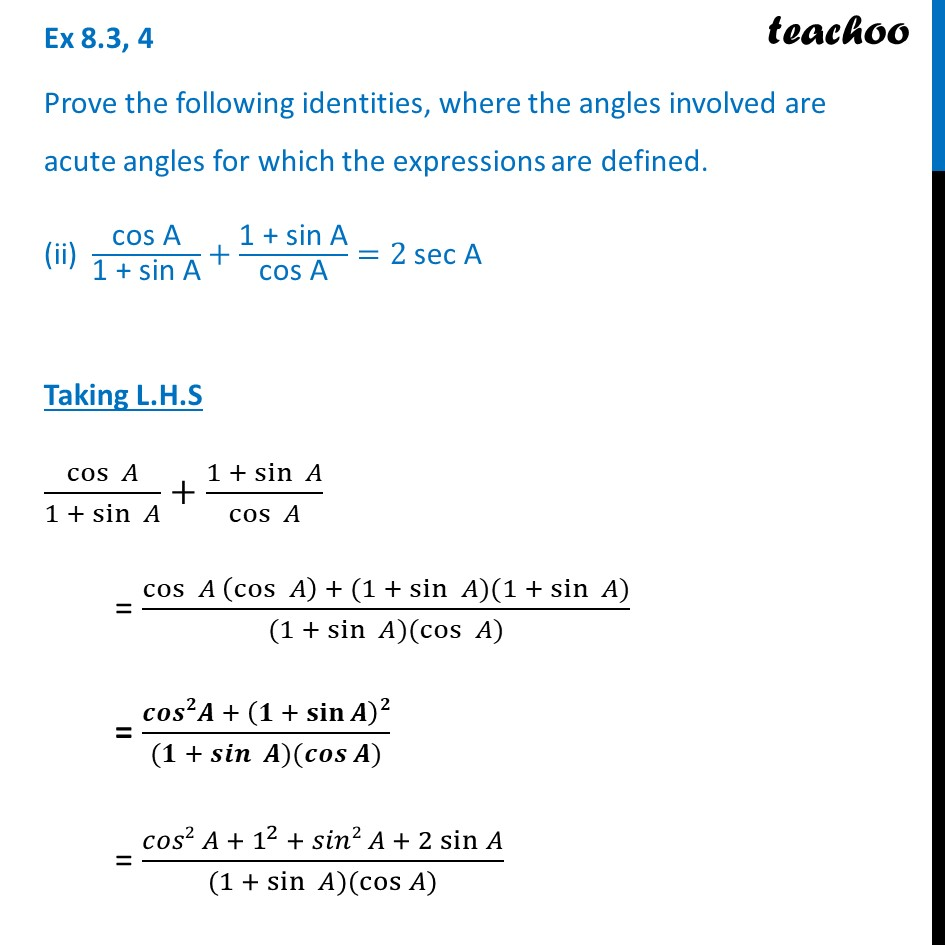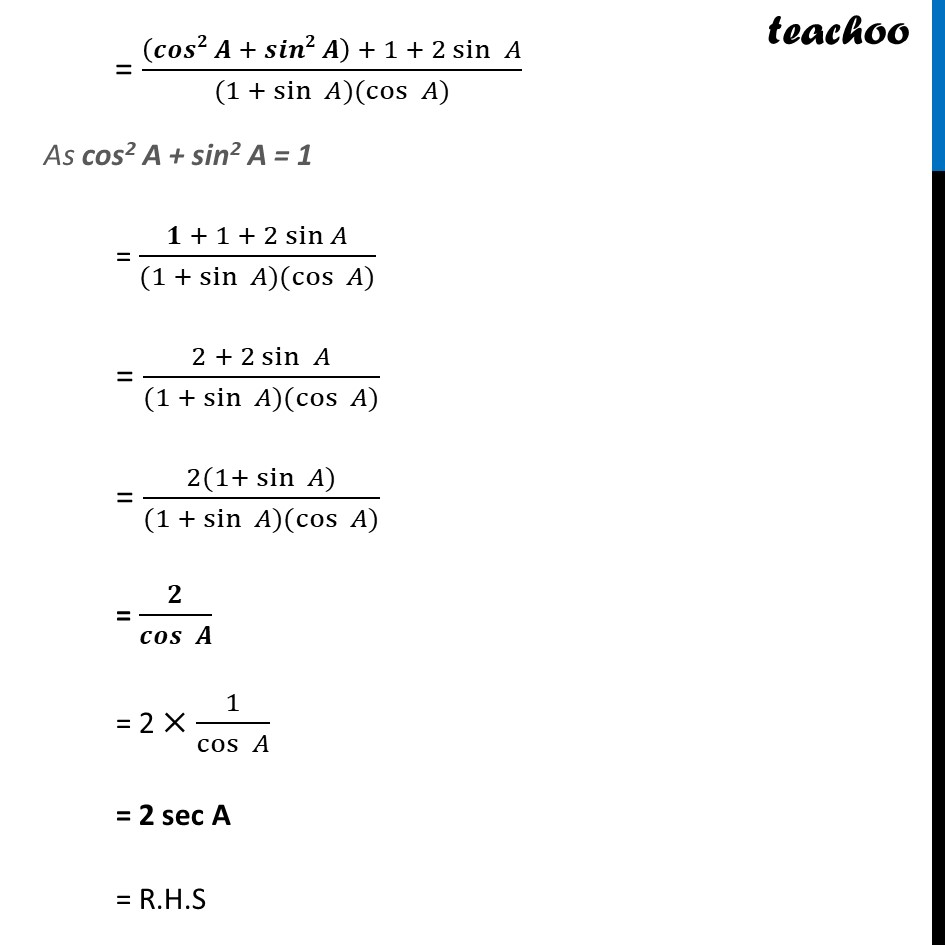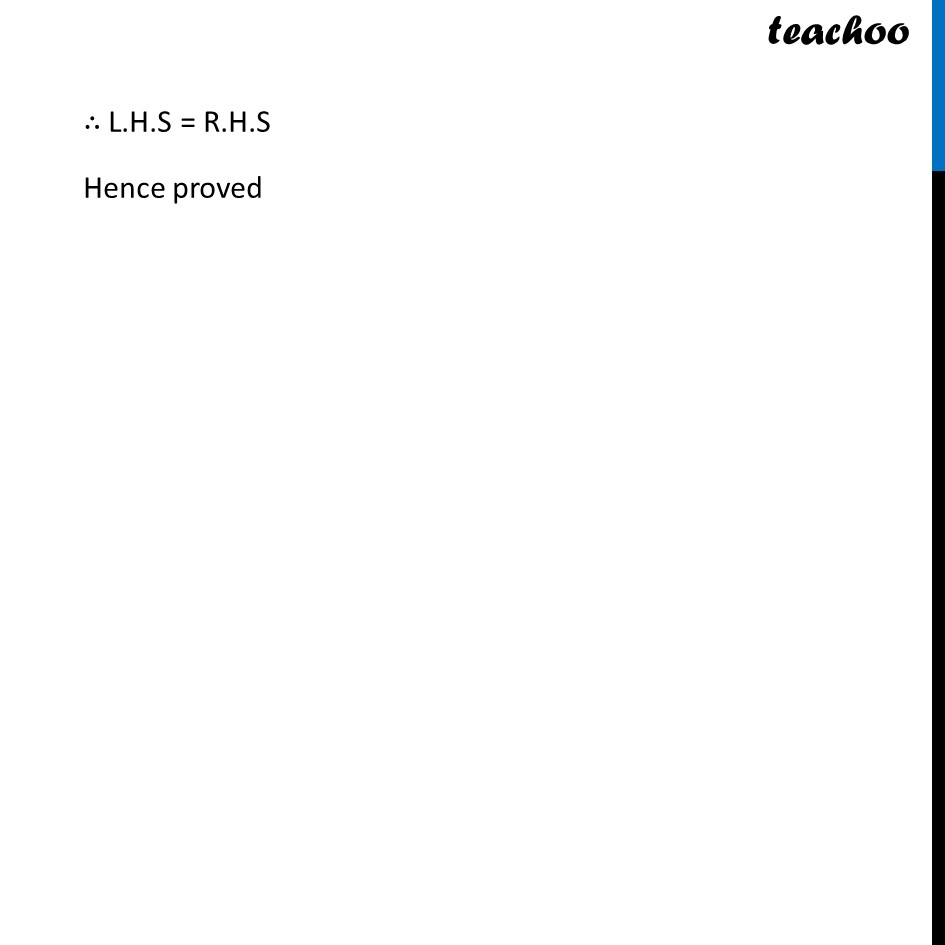Ex 8.3

Chapter 8 Class 10 Introduction to Trignometry
Serial order wiseLearn in your speed, with individual attention - Teachoo Maths 1-on-1 Class

### Transcript

Ex 8.3, 4 Prove the following identities, where the angles involved are acute angles for which the expressions are defined. (ii) "cos A" /"1 + sin A" +"1 + sin A" /"cos A" =2 sec A Taking L.H.S (cos⁡ 𝐴)/(1 + sin⁡〖 𝐴〗 )+(1 + sin⁡ 𝐴)/(cos⁡ 𝐴) = (cos⁡ 𝐴 (cos⁡ 𝐴) + (1 + sin⁡ 𝐴)(1 + sin⁡〖 𝐴)〗)/((1 + sin⁡ 𝐴)(cos⁡ 𝐴)) = (𝒄𝒐𝒔𝟐𝑨 + (𝟏 + 𝐬𝐢𝐧⁡𝑨 )𝟐)/((𝟏 + 𝒔𝒊𝒏⁡ 𝑨)(𝒄𝒐𝒔⁡𝑨)) = (𝑐𝑜𝑠2 𝐴 + 1^2 + 𝑠𝑖𝑛2 𝐴 + 2 sin⁡𝐴)/((1 + sin⁡ 𝐴)(cos⁡𝐴)) = ((𝒄𝒐𝒔𝟐 𝑨 + 𝒔𝒊𝒏𝟐 𝑨) + 1 + 2 sin⁡ 𝐴)/((1 + sin⁡〖 𝐴)(cos⁡ 𝐴)〗 ) As cos2 A + sin2 A = 1 = (𝟏 + 1 + 2 sin⁡𝐴)/((1 + sin⁡〖 𝐴)(cos⁡ 𝐴)〗 ) = (2 + 2 sin⁡ 𝐴)/((1 + sin⁡〖 𝐴)(cos⁡ 𝐴)〗 ) = (2(1+ sin⁡ 𝐴))/((1 + sin⁡〖 𝐴)(cos⁡ 𝐴)〗 ) = 𝟐/(𝒄𝒐𝒔⁡ 𝑨) = 2 ×1/cos⁡〖 𝐴〗 = 2 sec A = R.H.S ∴ L.H.S = R.H.S Hence proved